Exercise 10: Introduction to estimating diversification rates

In this tutorial, we will use phytools to create lineage-through-time (LTT) plots, compute the γ test statistic of Pybus & Harvey (2010), and then fit models of speciation & extinction.

Lineage through time plots

To get started, let's load phytools & dependencies:

``````library(phytools)
``````

Next, let's load a tree from file:

``````darter.tree<-read.tree("etheostoma_percina_chrono.tre")
``````

Our tree is from Near et al. (2011). In it, they sampled 201 of 216 species from the group (93%). For the purposes of our LTT plots and γ calculation, we will assume 100% sampling; however, we can relax this assumption subsequently.

Let's first plot the tree. All the taxon labels start with `"Percidae_"` and end with `"_2011"`. For our purposes this seems unnecessary, so let's remove them:

``````tip.label<-darter.tree\$tip.label
tip.label<-strsplit(tip.label,"_")
darter.tree\$tip.label<-sapply(tip.label,function(x) paste(x[2:3],collapse="_"))
plotTree(darter.tree,ftype="i",fsize=0.4,type="fan",lwd=1,part=0.88)
h<-max(nodeHeights(darter.tree))
obj<-axis(1,pos=-2,at=h-c(0,5,10,15,20),cex.axis=0.5,labels=FALSE)
text(obj,rep(-5,length(obj)),h-obj,cex=0.6)
text(mean(obj),-8,"time (mybp)",cex=0.8)
``````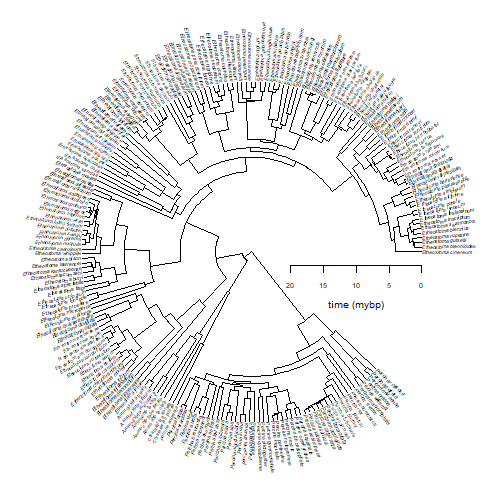Now let's use the function `ltt` in the phytools package to generate a LTT plot:

``````obj<-ltt(darter.tree,log.lineages=FALSE,col="blue",lwd=2)
``````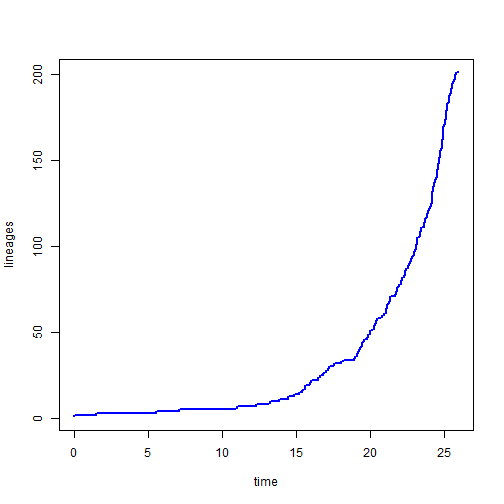``````obj
``````
``````## Object of class "ltt" containing:
##
## (1) A phylogenetic tree with 201 tips and 198 internal nodes.
##
## (2) Vectors containing the number of lineages (ltt) and branching times (times) on the tree.
##
## (3) A value for Pybus & Harvey's "gamma" statistic of NA, p-value = NA.
``````

This is weird. Well, it turns out that our tree is not fully bifurcating, so we need to resolve polytomies to continue:

``````darter.tree
``````
``````##
## Phylogenetic tree with 201 tips and 198 internal nodes.
##
## Tip labels:
##  Etheostoma_cinereum, Etheostoma_blennioides, Etheostoma_gutselli, Etheostoma_rupestre, Etheostoma_blennius, Etheostoma_swannanoa, ...
##
## Rooted; includes branch lengths.
``````
``````darter.tree<-multi2di(darter.tree)
darter.tree
``````
``````##
## Phylogenetic tree with 201 tips and 200 internal nodes.
##
## Tip labels:
##  Etheostoma_cinereum, Etheostoma_blennioides, Etheostoma_gutselli, Etheostoma_rupestre, Etheostoma_blennius, Etheostoma_swannanoa, ...
##
## Rooted; includes branch lengths.
``````
``````obj<-ltt(darter.tree,plot=FALSE)
obj
``````
``````## Object of class "ltt" containing:
##
## (1) A phylogenetic tree with 201 tips and 200 internal nodes.
##
## (2) Vectors containing the number of lineages (ltt) and branching times (times) on the tree.
##
## (3) A value for Pybus & Harvey's "gamma" statistic of 0.2007, p-value = 0.841.
``````
``````plot(obj,log.lineages=FALSE,main="LTT plot for darters",col="blue",lwd=2)
``````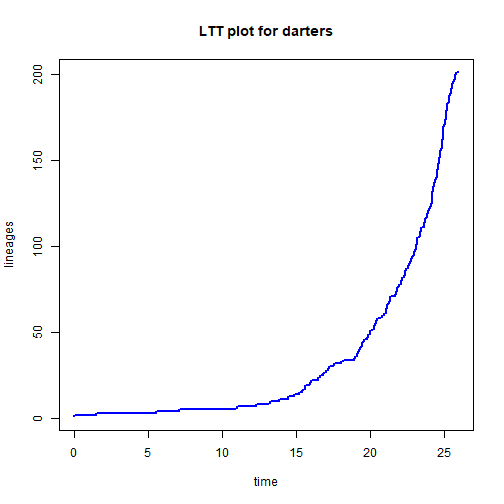It is even possible to visualize the LTT plot with the tree overlain:

``````plot(obj,show.tree=TRUE,log.lineages=FALSE,main="LTT plot for darters",lwd=2)
``````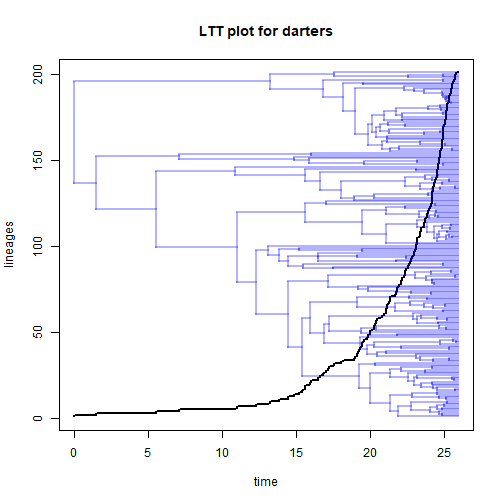Note that under pure-birth, the number of lineages should rise exponentially through time. By convention, and because it linearizes the expectation under pure-birth, we normally would plot our pure-birth trees on a semi-log scale, meaning that the vertical axis is on a log-scale while our horizontal axis is on a linear scale.

``````plot(obj,log.lineages=FALSE,log="y",main="LTT plot for darters",
ylim=c(2,Ntip(darter.tree)))
## we can overlay the pure-birth prediction:
x<-seq(0,h,by=h/100)
b<-phytools:::qb(darter.tree)
lines(x,2*exp(b*x),col="blue",lty="dashed",lwd=2)
``````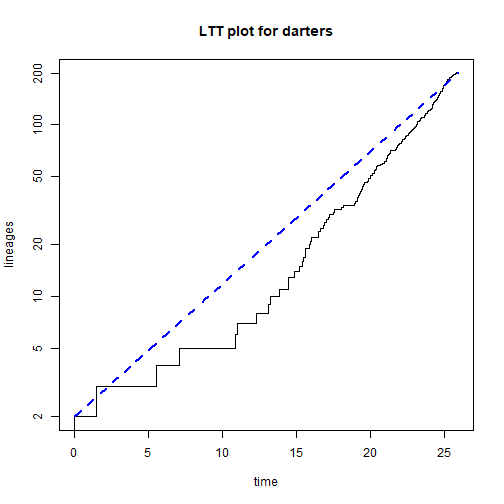We might like to compare the observed LTT, to simulated LTTs assuming a pure-birth process of the same duration & resulting in the same total number of species. We can do this using the phytools function `pbtree`:

``````trees<-pbtree(b=b,n=Ntip(darter.tree),t=max(nodeHeights(darter.tree)),
nsim=100,method="direct",quiet=TRUE)
obj<-ltt(trees,plot=FALSE)
plot(obj,col="grey",main="LTT of darters compared to simulated LTTs")
## now let's overlay our original tree
``````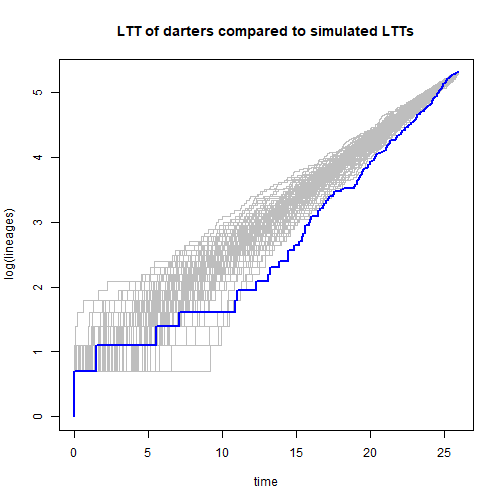``````## Object of class "ltt" containing:
##
## (1) A phylogenetic tree with 201 tips and 200 internal nodes.
##
## (2) Vectors containing the number of lineages (ltt) and branching times (times) on the tree.
##
## (3) A value for Pybus & Harvey's "gamma" statistic of 0.2007, p-value = 0.841.
``````

Estimating the speciation & extinction rates from the phylogeny of darters

Over 20 years ago Sean Nee et al. (1994) developed a statistical approach for estimation speciation & extinction rates from reconstructed phylogenies. Although other approaches have since been proposed, this continues to form the basis for the overwhelming majority of diversification models analyzed using reconstructed trees.

This model has been implemented in the phytools function `fit.bd`.

Let's fit the model to our darter tree from Near et al. (2011):

``````bd.model<-fit.bd(darter.tree)
bd.model
``````
``````##
## Fitted birth-death model:
##
## ML(b/lambda) = 0.2362
## ML(d/mu) = 0.015
## log(L) = 370.8757
##
## Assumed sampling fraction (rho) = 1
##
## R thinks it has converged.
``````

`fit.bd` can explicitly take into consideration incomplete sampling fraction following Stadler (2012). This is done with the argument `rho`. In our case we have sampled 201 or 216 species in this tree, a sampling fraction of:

``````sampling.f<-201/216
sampling.f
``````
``````##  0.9305556
``````

Let's fit our model:

``````bd.model<-fit.bd(darter.tree,rho=sampling.f)
bd.model
``````
``````##
## Fitted birth-death model:
##
## ML(b/lambda) = 0.2538
## ML(d/mu) = 0.0326
## log(L) = 370.8757
##
## Assumed sampling fraction (rho) = 0.930555555555556
##
## R thinks it has converged.
``````

Notice that the estimated extinction rate, μ, increases when we take incomplete sampling into account. Why?

Finally, we can also fit & compare a 'Yule' or pure-birth model as follows:

``````yule.model<-fit.yule(darter.tree,rho=sampling.f)
yule.model
``````
``````##
## Fitted Yule model:
##
## ML(b/lambda) = 0.2375
## log(L) = 370.7178
##
## Assumed sampling fraction (rho) = 0.930555555555556
##
## R thinks it has converged.
``````
``````AIC(yule.model,bd.model)
``````
``````##            df       AIC
## yule.model  1 -739.4357
## bd.model    2 -737.7514
``````

This suggests that (controlling for the number of parameters), a pure-birth (zero extinction) model fits better than a birth-death model for these data.

If we'd prefer, we can also easily conduct a likelihood-ratio (LR) test. For this we should install the package `lmtest`:

``````library(lmtest)
``````
``````## Loading required package: zoo
``````
``````##
## Attaching package: 'zoo'
``````
``````## The following objects are masked from 'package:base':
##
##     as.Date, as.Date.numeric
``````
``````lrtest(yule.model,bd.model)
``````
``````## Likelihood ratio test
##
## Model 1: yule.model
## Model 2: bd.model
##   #Df LogLik Df  Chisq Pr(>Chisq)
## 1   1 370.72
## 2   2 370.88  1 0.3157     0.5742
``````

Although the test is very simple so it would be straightforward to undertake “manually” as follows:

``````LR<--2*(logLik(yule.model)-logLik(bd.model))
LR
``````
``````##       202
## 0.3156765
## attr(,"df")
##  1
``````
``````P<-pchisq(LR,df=attr(LR,"df"),lower.tail=FALSE)
P
``````
``````##       202
## 0.5742176
## attr(,"df")
##  1
``````

Diversification models are also implemented in several other packages, including ape and in the powerful package diversitree. The following quickly shows how we would fit the same birth-death model using diversitree. It involves two steps - first we create the likelihood function (with `make.bd`), and then we fit it (using `find.mle`) as follows:

``````library(diversitree)
bd<-make.bd(darter.tree,sampling.f=sampling.f)
fitted.bd<-find.mle(bd,x.init=c(0.1,0.05),method="optim",lower=0)
fitted.bd
``````
``````## \$par
##     lambda         mu
## 0.25381855 0.03257651
##
## \$lnLik
##  370.8757
##
## \$counts
##       13       13
##
## \$convergence
##  0
##
## \$message
##  "CONVERGENCE: REL_REDUCTION_OF_F <= FACTR*EPSMCH"
##
## \$optim.method
##  "L-BFGS-B"
##
## \$method
##  "optim"
##
## \$func.class
##  "bd"       "dtlik"    "function"
##
## attr(,"func")
## Constant rate birth-death likelihood function:
##   * Parameter vector takes 2 elements:
##      - lambda, mu
##   * Function takes arguments (with defaults)
##      - pars: Parameter vector
##      - condition.surv [TRUE]: Condition likelihood on survial?
##      - intermediates [FALSE]: Also return intermediate values?
##   * Phylogeny with 201 tips and 200 nodes
##      - Taxa: Etheostoma_cinereum, Etheostoma_blennioides, ...
##   * Reference:
##      - Nee et al. (1994) doi:10.1098/rstb.1994.0068
## R definition:
## function (pars, condition.surv = TRUE, intermediates = FALSE)
## attr(,"class")
##  "fit.mle.bd" "fit.mle"
``````

We should see that the fitted parameter estimates are highly similar, if not identical, to those obtained from `fit.bd`. diversitree is a powerful R library that also includes a wide range of models that we will not cover in this short course, for instance permitting the pace of diversification to vary as a function of a discrete or continuous character trait (e.g., Maddison et al. 2007). The syntax for model-fitting, however, is highly similar to what we see in our simpler case.

Written by Liam J. Revell. Last updated 29 July 2018.